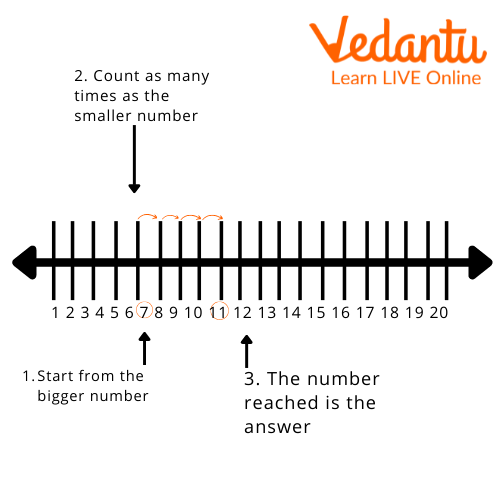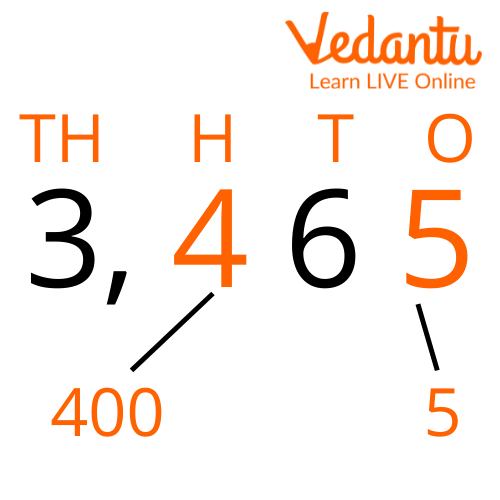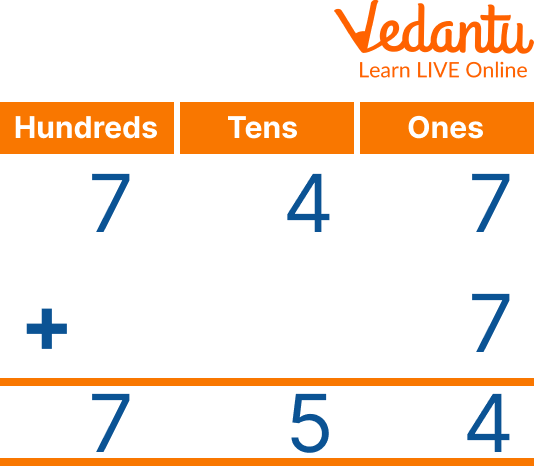Courses
Courses for Kids
Free study material
Free LIVE classes
MoreLIVE
Join Vedantu’s FREE Mastercalss

If you bought fifty candies in the morning and thirty-six more at night, then what is the total number of candies you bought in a day? Do you know the answer to this question? Let us learn the concept of addition for class 3 with questions and various examples.

The basic concept of addition was taught in the previous classes. In addition for class 3, we will learn a little advanced concept of addition. We will see how to do two-digit add and three-digit add. We will also understand the concept of addition with carrying. We will be looking at the concept with various additional examples. Addition questions will also be provided at the end. Maths worksheets for class 3 will also be shared at the end. So, let us begin the module now.

The addition is the sum of two or more numbers, be it single digit, double-digit, triple-digit, or so on. We can add any two numbers together and see the outcome of adding two numbers.

For Example, there are 7 strawberries in one basket and 4 strawberries in another basket. What would be the total number of strawberries?

We can understand this by this diagram where it is given that first take 7 on the line and then from 7 add 4 more. The result of the addition would be 11. This picture makes the concept simple and understandable.We can write this as 7 + 4 = 11 or seven + four = eleven. This is read as 7 plus 4 equals 11 in total. The addition of these can simply be done by using one’s fingers also.

Firstly, remember the digit 7 in your head and make 4 in your hand with the help of your fingers. As 7 is in your head, start counting 4 from 7 which is 8, 9, 10, and 11. Stop after counting 4 to 7 which would give you the result as 11.

Vertical addition is a method of adding where the numbers are lined up in columns according to their place values. The addition can be done vertically also. Let us see how to solve addition questions vertically. Firstly, let's learn the concept of place value.Place Value

The digit which is placed at the rightmost is called Ones because it is at one's place, the digit left to the Ones is called Tens because it is at tens place, and the digit left to Tens is called Hundreds. After all, it is a hundred places and the digit left to Hundred is called Thousands and so on. In the example given above, 5 is Ones, 6 is Tens, 4 is Hundreds, and 3 is a Thousand places.Look at the example of vertical addition. Here firstly, we have to add the digits in one place i.e. 7+7 which is equal to 14. As per the rule 4 is the digit at one's place, so write 4 in the one's box. Put the 1 of 14 as a carry to 4, then we have to add 4+1 which is 5. 5 will come in tenth place. Finally, the seven at the hundredth place would remain as 7.

Hence, the total would be 754.

## Solved Examples

Q 1. The first question is 459 + 587 = ?

Ans: By placing them in the order of place value, the addition can be done by the simple method. Firstly arrange them in a vertical format where the larger number is at the top. Start adding the ones digit then tens and so on. 9+7 is 16 so 1 would go as a carry-on 8. 8 would become 9 and then 9 would be added to 5. The answer to this would be 1046.

Q 2. What would be the sum of 766 + 235 ?

Ans: By placing them in the order of place value, the addition can be done by the simple method. Firstly arrange them in a vertical format where the larger number is at the top. Start adding the ones digit then tens and so on. 6+5 would be 11, then 1 would go as a carry-on 6 at tens place. 7+3 would be 10 so 1 would go as a carry-on 7. The answer to this would be 1001.

Q 1. 879 + 55 = ?

Ans: 934

Q 2. 777 + 666 = ?

Ans: 1443

## Summary

The addition for class 3rd is a little advanced and has to be understood well, all the concepts have to be understood at the base level only. It makes all the concepts of the future easy. These examples and methods have to be grasped well. Kids should solve as many maths worksheets as possible. Practice makes a man perfect. Here you will get new tricks for addition. This will make your studies fun. You can do addition in very less time after learning these rules.

Last updated date: 19th Sep 2023
Total views: 110.4k
Views today: 3.10k

## FAQs on Addition for Class 3

1. How do you solve the addition with two or more digits?

The addition method to perform the addition question of two or more digits is the same. Firstly we have to arrange the digits in place value order. All the digits should be correctly placed at ones, tenth, hundredth and thousandth values. And addition is always started from the right side by adding one's digits. Then after the ones, digits at the tenth place are added then digits at the hundredth place are added and finally, the digits at the hundredth value are added. The result of the addition is written below the question.

2. How to solve the addition in the number line?

The number line method for addition can also be done easily. Firstly a line has to be drawn with 10 dots under which one to 10 counting has to be written. Now say for example we have to add 2+4, the first mark 2 on the line. After that mark four more points from 2. The dot would reach 6 numbers on the line. This is the outcome. As 2 + 4 = 6. This can also be written as 2 plus 4 equals 6.

3. How does the addition of numbers help kids in their daily lives?

Addition as a concept is really important for kids to understand. It helps them master the art of numbers.

• It is used to total two numbers or the number of things. Whenever we go to shops to buy daily things, we usually add or subtract money.

• Apart from dealing with money, we also add various things like fruits in a dozen and distance in kilometers.

• In addition, it makes the base level of students very strong. It helps them to understand the advanced concepts related to addition.